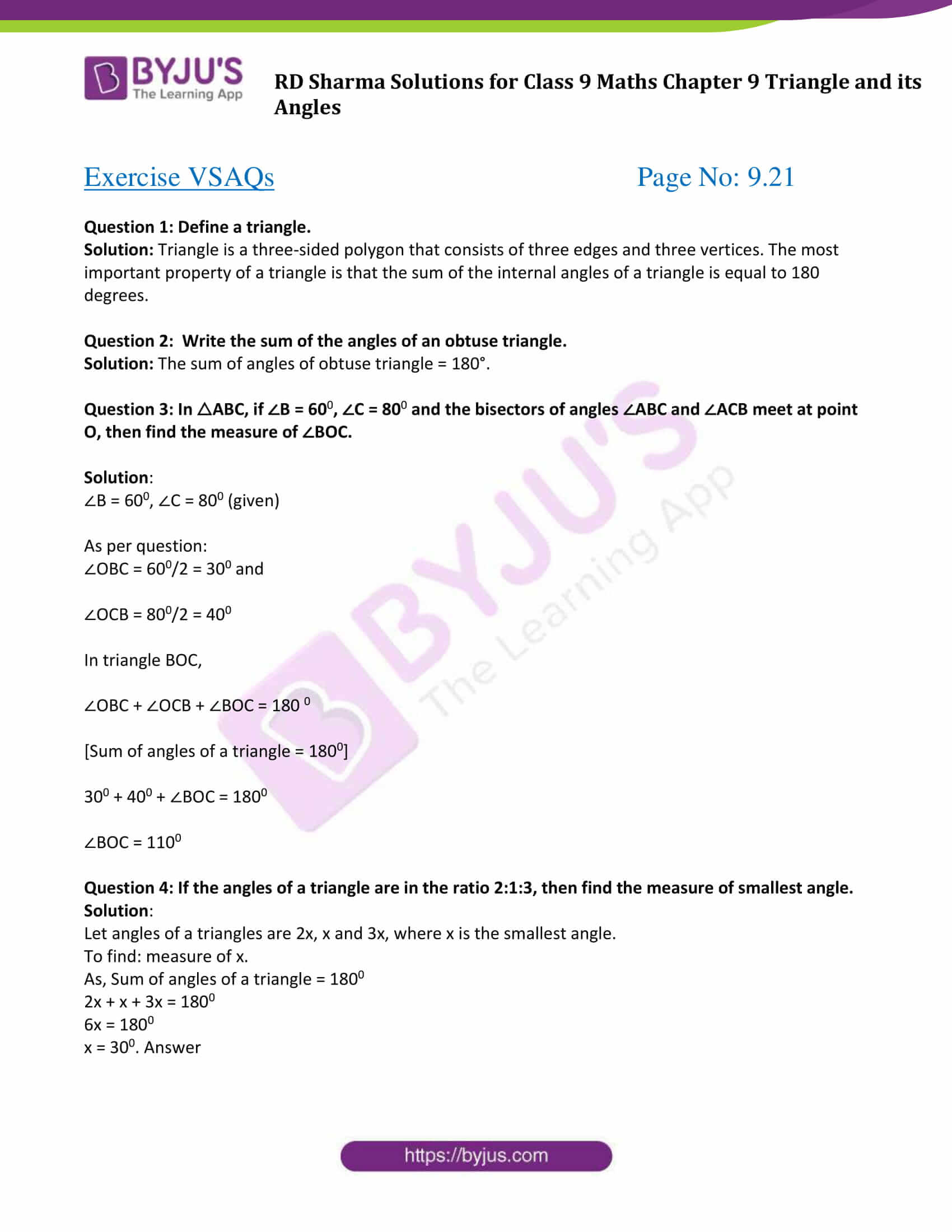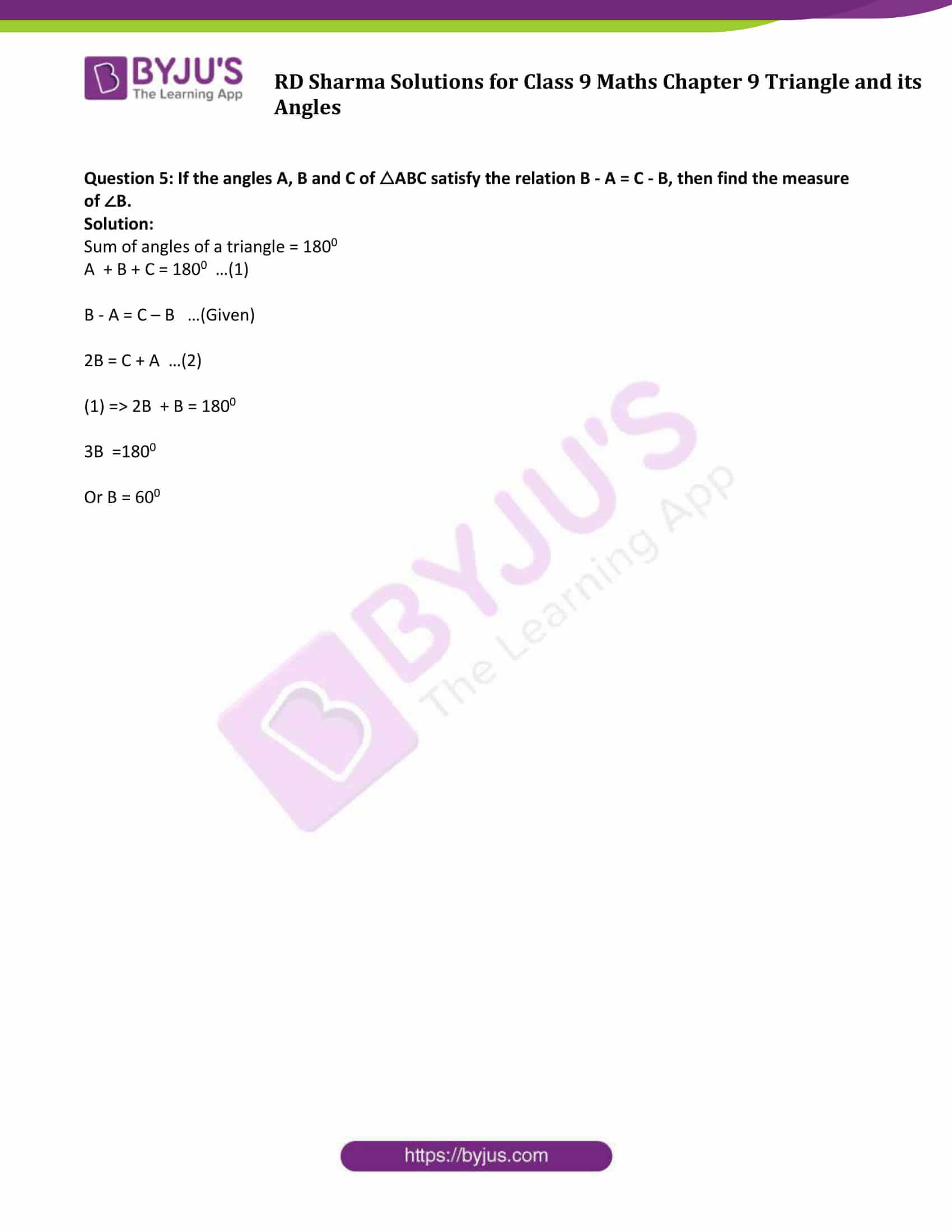# RD Sharma Solutions for Class 9 Maths Chapter 9 Triangle and its Angles Exercise VSAQs

RD Sharma Solutions for Class 9 Maths Chapter 9 exercise VSAQs is available here. Learn various concepts of triangles easily by practicing the questions from the exercises given in class 9 RD Sharma solutions for the chapter “Triangle and its Angles”. Students should practice problems on various concepts through solved RD Sharma solutions to have a better understanding.

## Download PDF of RD Sharma Solutions for Class 9 Maths Chapter 9 Triangle and its Angles Exercise VSAQs### Access Answers to Maths RD Sharma Solutions for Class 9 Chapter 9 Triangle and its Angles Exercise VSAQs Page number 9.21

Question 1: Define a triangle.

Solution: Triangle is a three-sided polygon that consists of three edges and three vertices. The most important property of a triangle is that the sum of the internal angles of a triangle is equal to 180 degrees.

Question 2: Write the sum of the angles of an obtuse triangle.

Solution: The sum of angles of obtuse triangle = 180°.

Question 3: In △ABC, if ∠B = 600, ∠C = 800 and the bisectors of angles ∠ABC and ∠ACB meet at point O, then find the measure of ∠BOC.

Solution:

∠B = 600, ∠C = 800 (given)

As per question:

∠OBC = 600/2 = 300 and

∠OCB = 800/2 = 400

In triangle BOC,

∠OBC + ∠OCB + ∠BOC = 180 0

[Sum of angles of a triangle = 1800]

300 + 400 + ∠BOC = 1800

∠BOC = 1100

Question 4: If the angles of a triangle are in the ratio 2:1:3, then find the measure of smallest angle.

Solution:

Let angles of a triangles are 2x, x and 3x, where x is the smallest angle.

To find: measure of x.

As, Sum of angles of a triangle = 1800

2x + x + 3x = 1800

6x = 1800

Question 5: If the angles A, B and C of △ABC satisfy the relation B – A = C – B, then find the measure of ∠B.

Solution:

Sum of angles of a triangle = 1800

A + B + C = 1800 …(1)

B – A = C – B …(Given)

2B = C + A …(2)

(1) => 2B + B = 1800

3B =1800

Or B = 600

## RD Sharma Solutions for Class 9 Maths Chapter 9 Triangle and its Angles Exercise VSAQs

Class 9 Maths Chapter 9 Triangle and its Angles Exercise VSAQs is helpful to revise all the concepts listed in this chapter. Students can download the pdf and clarify their doubts. RD Sharma Class 9 Chapter 9 helpful for students to understand the classifications of a triangle and some important and useful concepts of triangles.